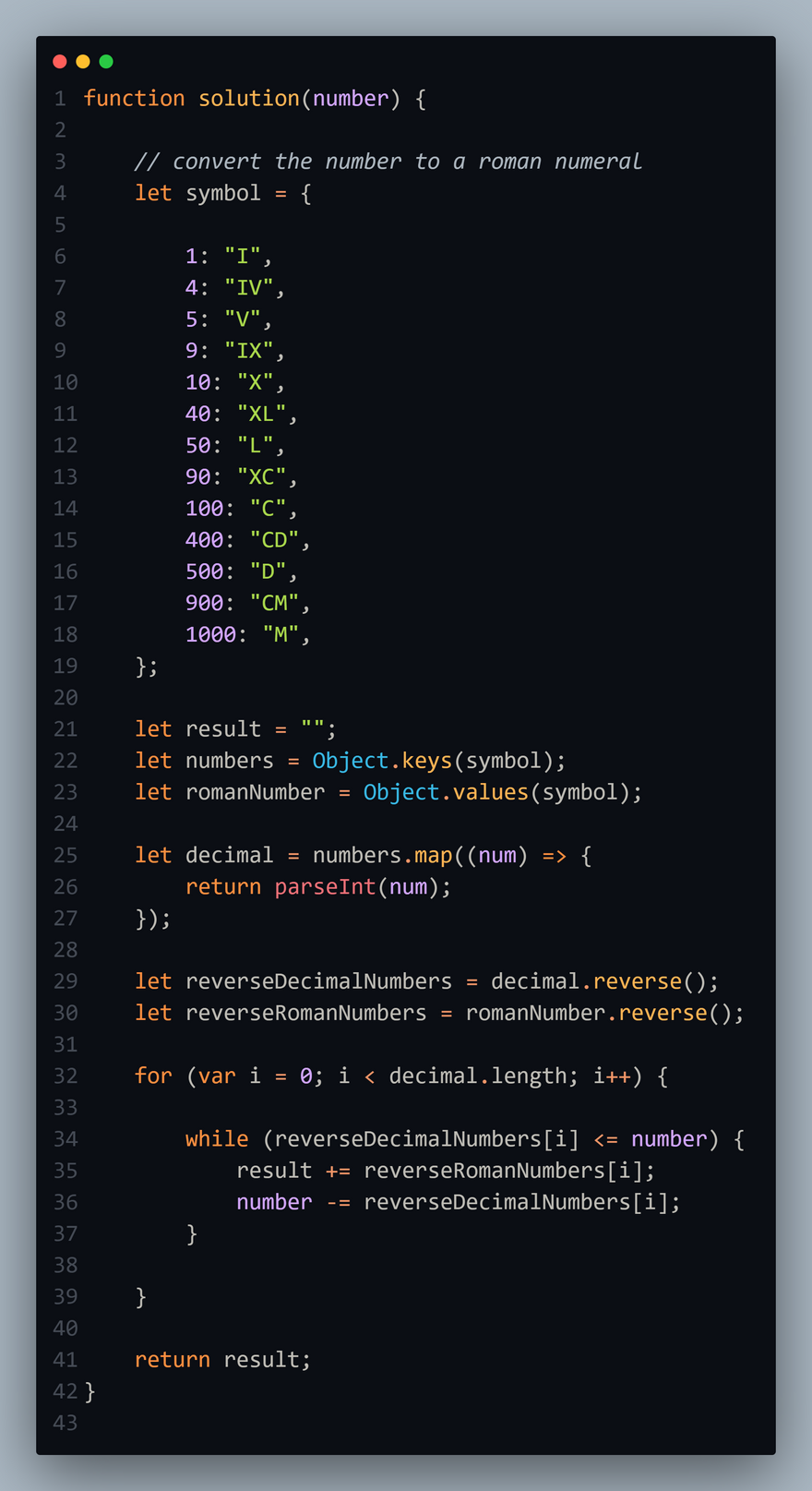## CodeNewbie Community 🌱# JavaScript 🐲 challenges_3 ⚔️

## Roman Numerals Encoder

• Create a function taking a positive integer as its parameter and returning a string containing the Roman Numeral representation of that integer.
• Modern Roman numerals are written by expressing each digit separately starting with the left most digit and skipping any digit with a value of zero.
• In Roman numerals 1990 is rendered: 1000=M, 900=CM, 90=XC; resulting in MCMXC.
• 2008 is written as 2000=MM, 8=VIII; or MMVIII.
• 1666 uses each Roman symbol in descending order: MDCLXVI.

#### Example:

• solution(1000); // should return 'M'

#### Help:

``````   Value    Symbol

1000:   'M',
900:    'CM',
500:    'D',
400:    'CD',
100:    'C',
90:     'XC',
50:     'L',
40:     'XL',
10:     'X',
9:      'IX',
5:      'V',
4:      'IV',
1:      'I'
``````

### My solution:

``````function solution(number) {

// convert the number to a roman numeral
let symbol = {

1: "I",
4: "IV",
5: "V",
9: "IX",
10: "X",
40: "XL",
50: "L",
90: "XC",
100: "C",
400: "CD",
500: "D",
900: "CM",
1000: "M",
};

let result = "";
let numbers = Object.keys(symbol);
let romanNumber = Object.values(symbol);

let decimal = numbers.map((num) => {
return parseInt(num);
});

let reverseDecimalNumbers = decimal.reverse();
let reverseRomanNumbers = romanNumber.reverse();

for (var i = 0; i < decimal.length; i++) {

while (reverseDecimalNumbers[i] <= number) {
result += reverseRomanNumbers[i];
number -= reverseDecimalNumbers[i];
}

}

return result;
}

``````

### Code Snapshot:🎥

### Connect with Me 😊